## The contents

The specific structure of the fixed-degree hypercubic networks implies that they route very well some permutations, but very badly some others. This is a general problem of many symmetric or recursive topologies. Parallel algorithms require often data rearrangements with regular patterns which may mismatch the regular and symmetric topology on networks.

In this section, we will treat hypercubic networks not only as direct networks, but also as multistage indirect networks (MIN), performing end-to-end permutations from the input nodes in the leftmost column to the output nodes in the rightmost column. The internal nodes are just switches. It is known that all the hypercubic MIN have equivalent end-to-end permutation routing properties. Without loss of generality, we may start with the ordinary butterfly to explain the main problems, and all the results mentioned here apply to all the hypercubic networks.
 Back to the beginning of the page Back to the CS838 class schedule

## On-line permutation routing

Let N=2n. Consider the following regular permutation patterns \pi:N-> N, defined as bitwise operations on n-bit binary strings.
• Bit-reversal \pib:un-1... u0-> u0... un-1.
• Transpose \pit:un-1... ukuk-1... u0-> uk-1... u0 un-1... uk if n=2k is even,
and \pit:un-1... uk+1ukuk-1... u0-> uk-1... u0ukun-1... uk+1 if n=2k+1 is odd.
• Translation by j, \pisj: i-> i \XOR j for a fixed n-bit string j.
• Complement \pic:un-1... u0->\ubn-1...\ub0 (special case of translation).

### Permutations with the worst-case greedy routing on oBFn

Lemma

Assume greedy on-line oblivious deterministic routing in all-port SF ordinary butterfly oBFn. Then the number of communication steps for permutations bit-reversal and transpose is \Theta(\sqrt(N)).
Proof
We will give the proof for the bit-reversal permutation, the argument for the transpose permutation is similar. Assume first that n is odd and let n=2k+1. Using greedy routing in oBFn, the packet from node (0,u) to (n,\pib(u)) is routed along the following path:CAPTION: Bit-reversal permutation greedy routing on end-to-end butterfly oBF3. The middle-stage edges have congestion 2.
(0,u)-> (1,un-1... u1un-1)-> (2,un-1... u2un-2un-1)->...->
(k,un-1... uk+1ukuk+1... un-1)-> (k+1,un-1... uk+1ukuk+1... un-1)->
(k+2,un-1... uk-1ukuk+1... un-1)->...-> (n,u0... un-1).

Let Uk=(k,un-1... uk+1ukuk+1... un-1), Uk+1=(k+1,un-1... uk+1ukuk+1... un-1), and ek=( Uk,Uk+1). We can easily observe that for given (k+1)-bit string un-1... uk, all packets sent from sources (0,un-1... uk *k) must pass through the channel corresponding to edge ek, since all these source nodes are leaves of CBTk with the root Uk. Let us call such a tree a left gather tree. The number of such packets is 2k=\sqrt(N/2), the first will cross ek in step k+1, and the last in step k+2k. This last packet then needs k steps to get into the rightmost column, so the lower bound on the number of steps of the routing algorithm is 2k+2k.

There are \sqrt(2N) left gather trees like this, each joined with the right half of the network via a single middle-stage edge, so that out of N middle-stage edges, only \sqrt(2N) are used for transferring N packets from the left to the right.

What are the memory requirements of an algorithm matching this lower bound? Assume \beta=O(2k). Consider any internal node in column i<= k of a left gather tree. It can accept 2 packets and send out one packet in one step, hence the largest accumulation of packets in its auxiliary buffers appears in step 2i-1 when all the routers in its left gather subtree has emptied their buffers, 2i-1 packets have gone, but 2i-1 are still stored. This holds for any i<= k, hence \beta <= 2k-1=\sqrt(N/8).

But it turns out that large buffers are not needed in fact, we can achieve the same lower-bound time with \beta=0. We just need a simple priority scheme to resolve the contention between two input packets over the single output channel to the parent router. For example, the packet with smaller source address has priority. If a packet moves up the tree, the whole chain of high priority packets from bellow moves one level up the tree. Consequently, the root of every left gather tree has in every step one packet to place on the middle-stage channel.

If n is even, the analysis is very similar and it gives nearly the same results --- the total number of steps is \sqrt(N)/2+n-1=\Theta(\sqrt(N)).

Hence, bit-reversal greedy permutation routing on a hypercubic topology performs equally badly with both large and constant buffers. The following lemma shows that the bit-reversal and the transpose permutations are (up to constant factors) examples of the worst-case permutations for greedy routing on hypercubic networks with nonconstant buffers.

Lemma

Let N=2n. Consider a routing problem in oBFn with at most one packet sent from each leftmost column node and at most one packet destined for each rightmost column node. If the channel buffer size is \Theta(\sqrt(N)), then any permutation can be routed in O(\sqrt(N)) steps using greedy algorithm.
Proof
Let ei be any edge of stage i and let gi be the number of greedy routes passing through ei. Clearly gi<= 2i, since ei cannot be reached from more than 2i input nodes of the corresponding left gather tree. Similarly, gi<= 2n-i-1, since at most 2n-i-1 output nodes can be reached from ei. Since a packet can be delayed at column i by at most other gi-1 packets that need to cross the same edge of stage i, the worst-case delay is \sumi=1n(gi-1). This sum is bounded by 3\sqrt(N/2)-n-2 if n is odd and by 2\sqrt(N)-n-2 if n is even.CAPTION: Worst-case time analysis of any permutation on oBFn.
Note that for small N=2n, say up to 128, there is almost no difference between \sqrt(N/2) and n=log N, so that problem becomes critical only for larger N. The worst-case permutations such as bit-reversal can be routed using precomputed instead of greedy routing (see the next subsection). Unfortunately, there are too many bad permutations for greedy routing so that we cannot precompute special routing for all of them.

### Permutations with optimal greedy routing on oBFn

On the other hand, many useful permutations can be routed on a store-and-forward all-port oBFn in O(n) communication steps using greedy routing. We will show two important representants.

#### Monotone routing

A routing is monotone (or order-preserving) if it does not change the relative order of the packets. For example, if N=8, then 1->2, 2->4, 5->6, 6->7 is a monotone routing, whereas 2->4, 3->1, 4->5, 7->6 is not. The most important example of a monotone routing is the packing problem. If we reverse the packing routing, we get spreading routing. By combining a packing with spreading, we can route any monotone routing.

Packing routing problem A packing problem consists of routing packets from any subset M of input nodes into the first |M| output nodes so that the relative order of the packets does not change. So, the i-th packet within M is destined for output node i (see Figure ). Since initially input nodes from M do not know anything about the other nodes with packets, they must first learn its relative order within M in order to compute the destination address. They can compute this information using a parallel prefix sum algorithm which will be described in Section #21. They need 2n communication steps for that. Assume every active node in M knows its destination.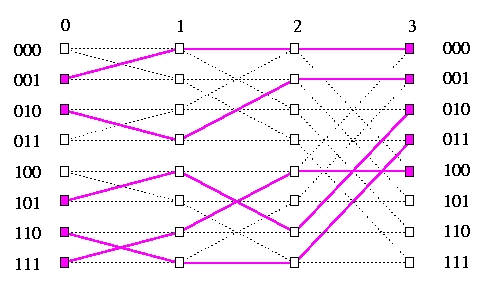CAPTION: Contention-free routing for a packing problem on oBF3.

Lemma

The greedy routing provides contention-free paths for any packing problem.
Proof
We show inductively that greedy routing provides packets with pairwise node-disjoint paths.
1. No two packets can collide on a node in column 1. Packets form any two source nodes which do not belong to the same stage-1 subbutterfly cannot collide. Consider two source nodes, say U0=(0,un-1... u10) and U1=(0,un-1... u11), sharing a stage-1 subbutterfly. Then they are consecutive in M and therefore they must end up in consecutive output nodes. Only two cases are possible.
• The packet from U0 will end up in some node (n,vn-1... v10). Then the packet from U1 will end up in node (n,vn-1... v11), which forces the greedy routing to use straight edges in the stage-1 subbutterfly for both packets.
• The packet from U0 will end up in some node (n,vn-1... v11). Then the packet from U1 will end up in node (n,v'n-1... v'10), which forces the greedy routing to use cross edges in the stage-1 subbutterfly for both packets.
In both cases, no link contention can appear in stage 1.
2. By induction, no link contention can appear on any subsequent stage. After passing the stage-1 links, let us partition the packets on those on odd-numbered horizontal rows and those on even-numbered horizontal rows.
• Any odd packet can never collide with an even packet, since the corresponding two subbutterflies are node-disjoint starting from stage 2.
• In each of these two subbutterflies, the same argument as in stage 1 applies.
We can conclude that any packing problem can be routed in the optimum number of steps. Packing problem appears in many parallel algorithms, in VLSI design, in some one-to-many collective communication problems. It can generalized so that the destination interval of |M| output nodes can have any offset from the top, it can even wrap-around the rightmost column. A special instance of a packing problem is a circular k-shift. We will see another application of the packing problem in radix sort.

Spreading routing problem and reduction of routing to sorting Spreading is the reverse of packing, we just move packets from right to left. A spreading problem consists of routing M=|M| packets from a contiguous set of nodes in column n to any any subset M of nodes in column 0 so that the relative order of the packets does not change. Greedy routing will provide a contention-free shortest paths in the same way as in the packing (see Figure ). The most important application of spreading is its use in reducing a general permutation to sorting.CAPTION: Contention-free routing for a spreading problem on oBF3.

Lemma

Any permutation can be routed on oBFn in time Ts(N)+O(n), where Ts(N) is the time to sort N items on oBFn.
Proof
Assume we are to route an arbitrary permutation on oBFn. If the number of packets is M=N, then we just sort them by destination addresses and use idempotent permutation (using only straight links). Assume M < N. After sorting, we have M packets packed in a contiguous subset of input nodes and we need just to apply the spreading to get them in a contention-free way to correct destinations. In Section # 17, we will see that Ts(N)=O(n2).

Monotone routing problem By combining one packing routing and one spreading routing, we can realize any monotone routing problem in O(n) steps on oBFn. A monotone routing is defined by giving an ordered set M1 of input nodes and ordered set M2 of output nodes, with |M1|=|M2|, such that the i-th node in M1 sends a packet to i-th node in M2. We perform packing of the packets from M1 by sweeping them left-to-right and then spreading by sweeping them right-to-left. If the packets are needed on the opposite side of oBFn, we transfer them using straight links. Figure shows monotone routing 0-> 2, 2-> 3,3->5,5->7 on oBF3.CAPTION: A simple example of monotone routing as a composition of packing and spreading routing.
Monotone routing has a variety of applications, e.g., in load balancing of parallel computations.

#### Translation and complement routing

Lemma

For any j, 0<= j<= N-1, the greedy routing provides node-disjoint paths for the translation permutation \pisj on oBFn, so that it is contention-free and takes n steps.
Proof
All the paths start in distinct input nodes. At each stage of oBFn, all the paths use either straight edges or cross edges. They can never content for an edge.CAPTION: Contention-free routing for translation by 3=011 on oBF3.
This argument becomes trivial in case of the complement permutation \pic defined on Page . Greedy routing guarantees the edge-disjointedness just because all the packets use only cross edges in all stages.

### Permutation routing on binary hypercube

All the greedy permutation routing algorithms on SF all-port end-to-end oBFn are normal hypercube algorithms. Therefore, they can be executed on any other hypercubic network and on shuffle-exchange and de Bruijn graphs with only a constant slowdown. Any contention-free permutation routing on oBFn provides a congestion-free permutation routing on wormhole full-duplex all-port Qn!!! Figure shows the correspondence, compare with Figure .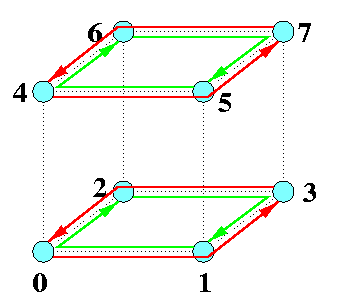CAPTION: Translation by 3=011 on full-duplex wormhole all-port Q3 using e-cube routing.
 Back to the beginning of the page Back to the CS838 class schedule

## Off-line permutation routing

Similarly to meshes, if a permutation is known beforehand globally, we can precompute off-line paths for routing such that the communication becomes contention-free and the permutation can routed in the optimal number of communication steps. Here, we show an elegant off-line algorithm that needs just 2n steps on an n-dimensional hypercubic network.

### Off-line permutation routing on a Benes network

The n-dimensional Benes network, denoted by BNn, is the n-dimensional back-to-back ordinary butterfly. We take oBFn and put its mirror copy on its right side so that the the last column of the butterfly and the first column of its mirror image merge. BNn has 2n+1 columns of nodes and 2n stages of edges. See Figure .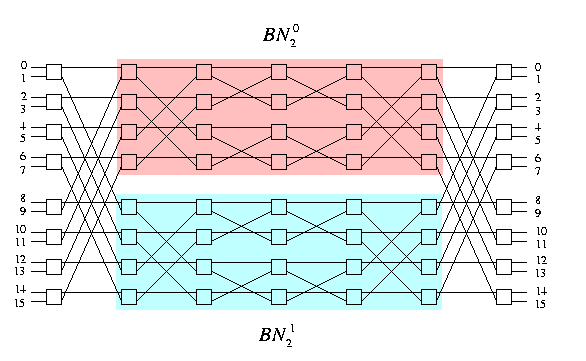CAPTION: 3-dimensional Benes network BN3.
Similarly to the ordinary butterfly, Benes network is hierarchically recursive. BNn contains two copies of BNn-1 as subgraphs, denoted as upper BNn-10 and lower BNn-11.

A key property of the Benes network is that it is rearrangeable complete network: for any permutation of inputs to outputs there is a contention-free routing. Let us state it formally in two lemmas.

Lemma

(Edge-disjoint routing.) Assume that each input/output node in BNn has two input/output channels, respectively. Let N=2n+1, N={0,...,N-1}, and let \pi:N->N be any permutation. Then in BNn, there is a set of N edge-disjoint paths joining input channels i to output channels \pi(i) for all iin\ Ns.CAPTION: Edge-disjoint paths in BN3 for the transpose permutation routing.
Proof
By induction on n, based on the hierarchical recursivity of BNn.

• Let us call two input (output) channels sharing an input (output, respectively) node twin channels Two channels are twins iff their least significant bits differ.
• Similarly, any two end-to-end paths i->\pi(i) and j->\pi(j) such that i and j are twin inputs (\pi(i) and \pi(j) are twin outputs) are called input (output, respectively) twin paths.
• Observe that each input node is linked by exactly one channel to the upper BNn-10 and by exactly one channel to the lower BNn-11.
• It follows that if a path uses the upper subnetwork, its input twin path must use the lower one, and vice versa. Symmetrically, the same holds for any pair of output twin paths, they must come from different subnetworks.
These observation justify the following algorithm which incrementally builds paths in a zig-zag way.
1. The lemma obviously holds for n=0.
2. Assume n>= 1 and assume it holds for n-1.
3. Take any iin\ Ns and without loss of generality, choose the upper subnetwork BNn-10 for forward routing the path i->\pi(i). This implies that we get to \pi(i) from the upper subnetwork.
4. Route the corresponding output twin path backward through the lower subnetwork and get back to the input column.
5. If the corresponding twin input path has been already decided, we have completed one cycle and we start with any unrouted path in the same way from step 3.
6. Otherwise, route corresponding input twin path forward through the upper subnetwork, and so on. We keep on going back and forth, using the upper subnetwork when going rightward and lower subnetwork when going backward until we close a cycle by getting into the starting input node.
7. After having decided the states of all switches in the leftmost and rightmost columns, we solve inductively induced permutations in BNn-10 and BNn-11.
Figure shows an edge-disjoint routing for the transpose permutation, which needs \Theta(\sqrt(N)) step on oBFn if greedy routing is used. Note that a given permutation has more edge-disjoint routings, the number of various solutions depends on n and the number of cycles in the routed permutation. If we reduce the number of input and output channels of the Benes network to one half, we can have another impressive result. Let BN'n denote the n-dimensional Benes network in which each input (output) node has only one input (output, respectively) channel. Assume that the inputs and outputs are numbered 0,...,2n-1 (see Figure ).

Lemma

Node-disjoint routing. Let N=2n, N={0,...,N-1}, and let \pi:N->N be any permutation. Then in BN'n, there is a set of node-disjoint paths, joining every input channel i to output channel \pi(i) for all iin\ Ns.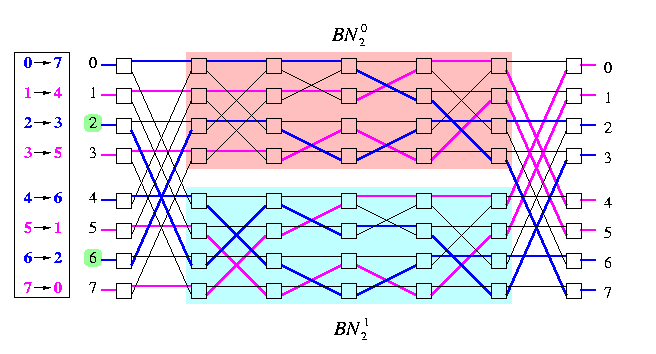CAPTION: Node-disjoint routing for a permutation on BN'3.
Proof
It is again an inductive proof similar to previous one, the only difference is in the definition of twins.

• Two input (output) nodes are called twins if they differ in the most significant bit. Define correspondingly twin paths.
• Observation: The node-disjointedness requires that if a path is routed through, say, the upper subnetwork, then both its input and its output twin paths must be routed through the lower subnetwork.
So the routing algorithm is identical with the previous one, except for a different definition of the relation is twin of.

### Off-line simulations of arbitrary topologies on oBFn

This property of the Benes network has several important implications, since
one pass through BNn = two passes forth and back in oBFn or wBFn.

Lemma

Let N=n2n and N={0,...,N-1}. Consider all-port full-duplex direct wBFn with at most one packet per processor. Then for any permutation \pi:N->N there exists an off-line deterministic permutation routing for \pi on wBFn which needs at most 3n communication steps and only constant buffers.
Proof
The routing algorithm is similar to the off-line permutation routing on 2D-meshes. We will view oBFn as M(2n,n) with 2n wrapped-around rows (= horizontal cycles of n nodes) and n vertical columns of 2n nodes. The algorithm has also 4 phases.
Phase 1:
Precompute permutations within horizontal cycles. Hence, apply Hall's matching theorem to n-regular routing bipartite graph with |X|=|Y|=2n vertices corresponding to source and destination cycle numbers to find n perfect matchings i=1,...,n specifying the set of packets to be aligned into column i of wBFn so that
all the destination cycle addresses of all packets in each vertical column become distinct.
Phase 2:
Perform these cycle permutations in all horizontal cycles in parallel. Trivial greedy routing needs n/2 steps for that.
In each column, there is at most one packet destined for any given horizontal cycle.
Phase 3:
Perform permutations of all columns in parallel to get all the packets into correct horizontal cycles. In contrast to M(2n,n), there are no vertical edges within the columns of wBFn, so that we have to simulate these permutations by applying the off-line node-disjoint routing algorithm on the Benes network.
1. Precompute off-line node-disjoint routing paths for each of these n column permutations by Lemma .
2. Since wBFn is vertex symmetric, the routing can start in all columns simultaneously and proceed in parallel by pipelining all these precomputed permutation waves synchronously in the same direction so that the total number of parallel communication steps is just 2n. Hence,
2a.
the waves move first synchronously in one direction from columns i to columns i+n n for all i,
2b.
then they move backward to column i by reversing directions.
The number of communication steps is 2n. After Phase 3,
all the packets are in correct horizontal cycles.
Phase 4:
Permute horizontal cycles so that the packets get into correct columns. This is again a trivial contention-free greedy routing which takes again only n/2 steps.
Figure sketches the phases of the routing algorithm.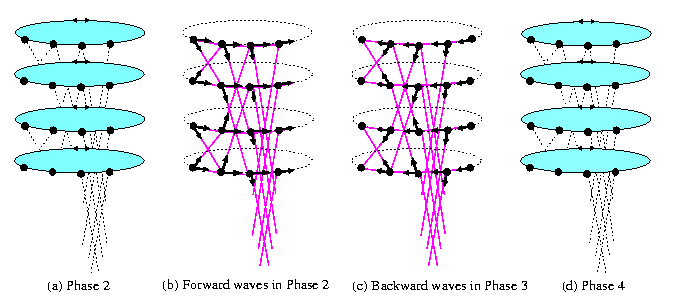CAPTION: Last three phases of the off-line deterministic permutation routing on wBF.
This result has several important applications.

Lemma

Let N=n2n. Let G be arbitrary N-node all-port full-duplex SF interconnection network with maximum node degree d. Then the direct all-port full-duplex SF wBFn can simulate G with slowdown O(dlog N) if precomputation is allowed. .CAPTION: Decomposition of a general communication step on an all-port full-duplex 4-node graph with maximum degree 3 into 3 permutations on wBF2.
Proof

• Since the topology of G is arbitrary, we apply an arbitrary one-to-one vertex mapping of V(G) onto V(wBFn).
• Since the node degree of G is bounded by d, each node in G can send at most d packets and receive at most d packets in one communication step. We simulate
one neighbor-to-neighbor global communication step on G
with
d permutation routings on wBFn
• This simulation is therefore a serialization: one global step is serialized into at most d substeps, when each node exchanges packets with only one neighbor.
• The permutations are not necessarily complete since G is not necessarily d-regular and all the nodes do not necessarily use all their channels in every communication step.
Figure gives an example for d=3.

Corollary

N-node all-port full-duplex wBFn can simulate N-node hypercube with slowdown O(log2N), if precomputation is allowed.
Proof
By previous lemma if G is the hypercube of size N. What is even more important, Lemma implies that the hypercube itself is able to simulate any bounded-degree topology optimally.

Corollary

N-node hypercube can simulate any (Nlog N)-node bounded-degree network G with slowdown O(dlog N), d being the maximum node-degree of G, if precomutation is allowed.
Proof
We know that by shrinking all the horizontal cycles of wBFn into nodes, we get hypercube Qn. So, 2n-node hypercube can simulate n2n-node wBFn with slowdown n, since one hypercube node has to simulate n nodes of the butterfly and the edges of Qn can simulate the edges of wBFn with only a constant slowdown.
 Back to the beginning of the page Back to the CS838 class schedule